# Java AWT | Ellipse2D

Ellipse2D class is present in java.awt.geom package and it is used to define an ellipse by stating its framing rectangle. This class is only the abstract superclass for all objects which store a 2D ellipse.

• Ellipse2D.Double defines an ellipse with double precision.
• Ellipse2D.Float defines an ellipse with float precision.

Constructor of the class are:

1. Ellipse2D.Double() : creates an ellipse at location 0, 0 and size 0, 0.
2. Ellipse2D.Double(double x, double y, double w, double h) : creates an ellipse at location x, y and width w and height h.
3. Ellipse2D.Float() : creates an ellipse at location 0, 0 and size 0, 0.
4. Ellipse2D.Float(Float x, Float y, Float w, Float h) : creates an ellipse at location x, y and width w and height h.

Commonly used methods:

method explanation
contains(double x, double y) returns whether the point is inside the ellipse or not
contains(double x, double y, double w, double h) returns whether the rectangle is inside the ellipse or not
equals(Object o) Determines whether or not the specified Object is equal to this Ellipse2D.
intersects(double x, double y, double w, double h) returns whether the rectangle intersects the ellipse or not
setFrame(double x, double y, double w, double h) Sets the location and size of the framing rectangle of this Shape to the specified rectangular values.

Below programs illustrate the Ellipse2D class:

1. Java program to create two ellipses and draw them to a java applet: To create ellipse shape on Java applet, we will initialize Ellipse2d class objects named “ed” and “ed1”. The 4 parameters passed in the constructor of the “ed” object are the X coordinate of the upper-left corner of the framing rectangle, the Y coordinate of the upper-left corner of the framing rectangle, the width of the framing rectangle and the height of the framing rectangle. In the constructor of “ed”, we pass nothing, which means the ellipse is initialized to location (0, 0) and size (0, 0). To show them on screen, we create an object of Graphics2d class “g1” and call g1.draw() method.

 `// java program to create two ellipse and  ` `// draw them to a java applet ` `import` `java.awt.*; ` `import` `javax.swing.*; ` `import` `java.awt.geom.*; ` `public` `class` `solve1 ``extends` `JApplet { ` `    ``public` `void` `init() ` `    ``{ ` `        ``setSize(``300``, ``300``); ` `    ``} ` ` `  `    ``// paint the applet ` `    ``public` `void` `paint(Graphics g) ` `    ``{ ` `        ``// create a ellipse2d ` `        ``Ellipse2D ed = ``new` `Ellipse2D.Double(``100``.0d, ``100``.0d,  ` `                                             ``120``.0d, ``80``.0d); ` ` `  `        ``// create another ellipse2d ` `        ``Ellipse2D ed1 = ``new` `Ellipse2D.Double(); ` ` `  `        ``// set framing rectangle of ellipse ` `        ``ed1.setFrame(``100``.0d, ``100``.0d, ``80``.0d, ``120``.0d); ` ` `  `        ``Graphics2D g1 = (Graphics2D)g; ` ` `  `        ``g1.setColor(Color.red); ` ` `  `        ``// draw the first ellipse ` `        ``g1.draw(ed); ` ` `  `        ``g1.setColor(Color.blue); ` ` `  `        ``// draw the first ellipse ` `        ``g1.draw(ed1); ` `    ``} ` `} `

Output: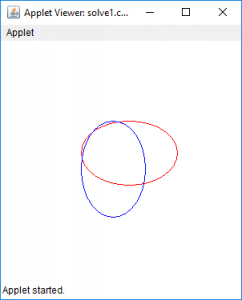2. Java program to create two ellipse and check whether a point or a rectangle is contained in that ellipse or intersected by it: To check whether a point or a rectangle is contained in that ellipse or intersected by the 2 ellipses, we first create the 2 ellipses in the similar way we created above. Then we create 2 rectangles, by calling the method drawRect() on Graphics2d object “g1”. The parameters in drawRect() method specify the x coordinate of the rectangle to be drawn, the y coordinate of the rectangle to be drawn, the width of the rectangle to be drawn and the height of the rectangle to be drawn. To check whether they contain it or not, we call ed.contains() method and pass the coordinate of the point or rectangle in it, and show the results on a message dialog.

 `// java program to create two ellipse and check whether ` `// a point or a rectangle is contained in that ellipse ` `// or intersected by it ` `import` `java.awt.*; ` `import` `javax.swing.*; ` `import` `java.awt.geom.*; ` `public` `class` `solve2 ``extends` `JApplet { ` `    ``public` `void` `init() ` `    ``{ ` `        ``setSize(``300``, ``300``); ` `    ``} ` ` `  `    ``// paint the applet ` `    ``public` `void` `paint(Graphics g) ` `    ``{ ` `        ``// create a ellipse2d ` `        ``Ellipse2D ed = ``new` `Ellipse2D.Double(``100``.0d, ``100``.0d, ` `                                             ``120``.0d, ``80``.0d); ` ` `  `        ``// create another ellipse2d ` `        ``Ellipse2D ed1 = ``new` `Ellipse2D.Double(); ` ` `  `        ``// set framing rectangle of ellipse ` `        ``ed1.setFrame(``100``.0d, ``100``.0d, ``80``.0d, ``120``.0d); ` ` `  `        ``Graphics2D g1 = (Graphics2D)g; ` ` `  `        ``g1.setColor(Color.red); ` ` `  `        ``// draw the first ellipse ` `        ``g1.draw(ed); ` ` `  `        ``g1.setColor(Color.blue); ` ` `  `        ``// draw the first ellipse ` `        ``g1.draw(ed1); ` ` `  `        ``g1.setColor(Color.black); ` ` `  `        ``// draw a rectangle ` `        ``g.drawRect(``100``, ``100``, ``80``, ``100``); ` ` `  `        ``g1.setColor(Color.orange); ` ` `  `        ``// draw a rectangle ` `        ``g.drawRect(``150``, ``150``, ``10``, ``10``); ` ` `  `        ``// does it contain point ` `        ``JOptionPane.showMessageDialog(``this``, ``"ellipse 1 contains point 150, 150  "`  `                                                        ``+ ed.contains(``150``, ``150``)); ` ` `  `        ``// does it contain rectangle ` `        ``JOptionPane.showMessageDialog(``this``, ``"ellipse 1 contains rectangle at"` `+ ` `        ``"  150, 150 of width 10 and height 10 "` `+ ed.contains(``150``, ``150``, ``10``, ``10``)); ` ` `  `        ``// does it contain rectangle ` `        ``JOptionPane.showMessageDialog(``this``, ``"ellipse 1 contains rectangle at "``+ ` `         ``" 150, 150 of width 80 and height 100 "` `+ ed.contains(``150``, ``150``, ``80``, ``100``)); ` ` `  `        ``// does it  intersect rectangle ` `        ``JOptionPane.showMessageDialog(``this``, ``"ellipse 1 intersect rectangle at "``+ ` `        ``" 150, 150 of width 80 and height 100 "` `+ ed.intersects(``150``, ``150``, ``80``, ``100``)); ` `    ``} ` `} `

Output: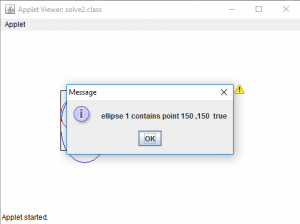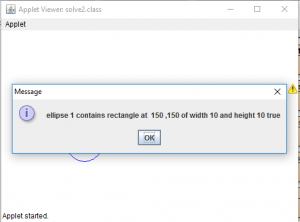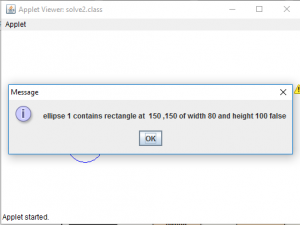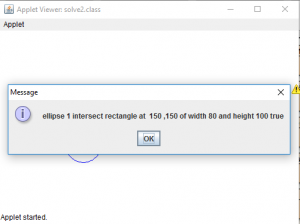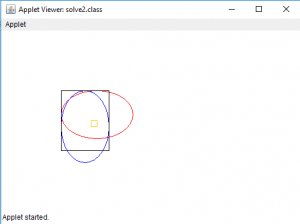Note: The above programs might not run in an online IDE please use an Offline compiler.

Don’t stop now and take your learning to the next level. Learn all the important concepts of Data Structures and Algorithms with the help of the most trusted course: DSA Self Paced. Become industry ready at a student-friendly price.

My Personal Notes arrow_drop_upCheck out this Author's contributed articles.

If you like GeeksforGeeks and would like to contribute, you can also write an article using contribute.geeksforgeeks.org or mail your article to contribute@geeksforgeeks.org. See your article appearing on the GeeksforGeeks main page and help other Geeks.

Please Improve this article if you find anything incorrect by clicking on the "Improve Article" button below.

Improved By : ManasChhabra2

Article Tags :
Practice Tags :

Be the First to upvote.

Please write to us at contribute@geeksforgeeks.org to report any issue with the above content.